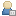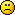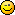###Show Posts

This section allows you to view all posts made by this member. Note that you can only see posts made in areas you currently have access to.

### Topics - Hemlos

Pages: 1 ... 8 9 
136
##### Beta Tests / GLBasic 3d Font Object Creator BETA: Closed
« on: 2008-Aug-07 »
Sorry this project is closed for now, unfortunetly the source is gone due to a crash.

You can still use the image2object creator for making similiar objects.
The source in image2object was the core code for this project.

This program did everything including circular words, and texture mapping it with 3 styles of overlay.
It stretched it evenly, or it tiled each letter with the map, or it stretched it to fit x y.
The texture was directly inputed into the vertice xyz points color data...no need for x_settexture.

The way it worked was it read in a smalfont.png, then with some math it built an object out of the text input.
It made a cube for each pixel in the sentance\$ input.
You were able to select a color for the object with a color map.
It was scalable, and it was able to make the words go into a circle, the font downside pointing inwards of the circle. And the circle was scalable too.

Making this object flat or thick with a scalar was possible...also if you made it 0 thickness..it was set to make shadows too.
All the 3d object creation options were available.

Im sad i lost this codeIf someone wants to try whipping up a new one....you may have the image2object code to do so freely.
Just let me know youre going to give it a shot, ill help you.

137
##### GLBasic - en / Sell and prevent sharing?
« on: 2008-Jul-31 »
I made a tool and i would like to sell it to people who use the game it is for.
I didnt make the game, only the tool.

1. How do i pack and sell the program so people cant copy it and share it?
2. How do i go about doing this legally, without infringing on the original developers rights?

Maybe these sound like stupid questions, but ive never actually considered this till now, because ive actually created something useful, and would like to profit from it....residual income would nice-Hem

138
##### 3D-snippets / 3D GRAVITY!
« on: 2007-Jul-11 »
June 14, 2012:
I attached a 3d galactic simulator to this message...play with the file called "Profile"...youll be able to affect the gravity and shape of the formations.
I made a bunch of different formations..these buttons will start them:
`1234567890-=[backspace]

I APPOLOGIZE from the bottom of my heart, really, im sorry for not keeping up with this thread.
It is sloppy, on the fly recoding, to keep up with the IDE...i have too much on my plate.
If you need help with your own program handling 3d gravity, i can help you by reviewing code.

Here is how you do 3d gravity...
Basically...i just used isaac newtons formula on each dimension, then divided by the number of dimensions.
You must alter the ALL forces on every object, which is induced by all other objects with mass.
In other words...if theres 100 objects...you must change the force applied to each object, 99 times(indices of all masses affect each one)
...and then do it for the second(third, fourth,etc) object...so this would be 100x99 loops(not 100x100 because you dont calculate the gravity of an object on itself X)

In order to explain the gravity, i ripped the most important code and splattered it here below as neatly as i could:
Code: (glbasic) [Select]
`//Predefine Gravity and masses:G=1.615 //Gravitational constant...this must not change. The value can be anything you want.//All objects must have predefined MASS(size), and Position.// In other words, one object might have the mass of 3 while another object can be size of 10.//so you begin with a loop calculating each object separately://You apply all the forces of all objects onto the first object.....then again for the second and third...etcM = mass of the object 1//xyz of object, which gravity force will be applied to:X=ObjPos[Object1] // xyz of Object(next in indices) being affectedY=ObjPos[Object1]Z=ObjPos[Object1]//this is the beginning of the SECOND loop...apply force to first object according to all the other forces in the indices//Define position of next objects force://Object #2 xyz position (next XYZ)nX=ObjPos[Object2] // xyz of Obj2 of next object in listnY=ObjPos[Object2]nZ=ObjPos[Object2]//vector distance(Range)://R must be > 1...i limited my test to 1.001 as a minimum..or else i forced a collision event here.//You will experience a [numerical terminal velocity] of objects colliding, when the vector distance drops to 1.0RX=(MAX(X,nX)-MIN(X,nX))RY=(MAX(Y,nY)-MIN(Y,nY))RZ=(MAX(Z,nZ)-MIN(Z,nZ))R2=(RX*RX)+(RY*RY)+(RZ*RZ)R=SQR(R2)R3=(R*R*R)//Now you must apply the gravity to the force being put on the first object(XYZ affected by xforce)// you keep altering this xforce until all indices are read.Gravity=G*M2 //this is force being applied to the current force...not really gravity..variables need names.IF X>nX THEN XForce=XForce-Gravity*RX/R3IF X<nX THEN XForce=XForce+Gravity*RX/R3IF Y>nY THEN YForce=YForce-Gravity*RY/R3IF Y<nY THEN YForce=YForce+Gravity*RY/R3IF Z>nZ THEN ZForce=ZForce-Gravity*RZ/R3IF Z<nZ THEN ZForce=ZForce+Gravity*RZ/R3//end of loop....by this point, first object will calculated, because you applied the forcee from all other objects on the first one//the loop will now run through and affect the force applied to the second object in the indices of objects.// after all objects are calculated//now you must move the object according to the forces applied onto it:ObjPos[Object1]=X+XForceObjPos[Object1]=Y+YForceObjPos[Object1]=Z+ZForce//and dont forget, you must record into memory the force applied onto the object you are moving here.//this data will be used again....in the next program frame.ObjPos[Object1]=XForceObjPos[Object1]=YForceObjPos[Object1]=ZForce`
I know..its hard to understand...im not a very good teacher, but someone might 'get it'.

139
##### 3D-snippets / X_MULTMATRIX
« on: 2006-Nov-06 »
Code: (glbasic) [Select]
`DIM matmat[0..3] = forward (local x-axis) (x,y,z,0)mat[4..7] = local up (x,y,z,0)mat[8..11]=local right (x,y,z,0)mat[12..16]=translation (offset) from origin (x,y,z,1)X_MULTMATRIX mat[]`If you want to use X_ROTATION and stuff, too - use the X_PUSHMATRIX before X_MULTMATRIX.

*quoted from gernot*

Quote
Note from Hemlos:
Im currently working with this new command and will update this in more detail as i get more information.

140
##### GLBasic - en / 3d rotations issue
« on: 2006-Oct-13 »
Sorry, my mail is not working so i have to post this bug report on the forums...

With each 3d object the heirarchy order of rotations are different.... the code is NOT universal. It doesnt work every object the same way! I added 3 x_popmatrix like you said, after the drawobj, but that doesnt help much... after  too many rotations, and after a while none of the rotations have been pushed correctly at all.

With almost a hundred hours of testing...i found the rotations dont truely stack, not matter how i code it, no matter how i place pushmatrix....in retrospect, x_movement pushes fine.

i suspect the problem is in the pushmatrix/popmatrix/rotation commands because 2 matrices do not "push" the stack the same way.
Theoratically in 3d you should only have to rotate any object a maximum of 3 times, if you push the stack 3 times, right? I ask because i need a 4th rotation for one of my objects, because 3 isnt sufficient.

141
##### 3D-snippets / Make Simple 3d Objects with functions.
« on: 2006-Oct-10 »
Here's some source code for simple 3D objects with textures.

A Sphere
Code: (glbasic) [Select]
`// ------------------------------------------------------------- //// -=#  SPHERE  #=-// ------------------------------------------------------------- //FUNCTION CreateSphere: num, r, n, colLOCAL i,j, theta1, theta2, theta3, pi pi = ACOS(0)*2 IF r < 0 THEN r = -r IF n < 3 THEN n = 3 X_OBJSTART num FOR j=0 TO n/2-1 theta1 = j * 2*pi / n - pi/2; theta2 = (j + 1) * 2*pi / n - pi/2; FOR i=0 TO n theta3 = i * 2*pi / n; X_OBJADDVERTEX r*COS(theta2) * COS(theta3), r*SIN(theta2), r*COS(theta2) * SIN(theta3), i/n, 2*(j+1)/n, col X_OBJADDVERTEX r*COS(theta1) * COS(theta3), r*SIN(theta1), r*COS(theta1) * SIN(theta3), i/n, 2*j/n, col NEXT X_OBJNEWGROUP NEXT X_OBJENDENDFUNCTION // n`A Cube - each side has the same texture
Code: (glbasic) [Select]
`// ------------------------------------------------------------- //// -=#  CREATECUBE  #=-// ------------------------------------------------------------- //FUNCTION CreateCube: num, sz, col // Diese Variablen sind als LOCAL definiert: // num, sz, sz=sz/2 X_OBJSTART num // Front Face X_OBJADDVERTEX  sz, -sz,  sz, 1, 0, col X_OBJADDVERTEX -sz, -sz,  sz, 0, 0, col X_OBJADDVERTEX  sz,  sz,  sz, 1, 1, col X_OBJADDVERTEX -sz,  sz,  sz, 0, 1, col X_OBJNEWGROUP // Back Face X_OBJADDVERTEX -sz,  sz, -sz, 1, 1, col X_OBJADDVERTEX -sz, -sz, -sz, 1, 0, col X_OBJADDVERTEX  sz,  sz, -sz, 0, 1, col X_OBJADDVERTEX  sz, -sz, -sz, 0, 0, col X_OBJNEWGROUP // Top Face X_OBJADDVERTEX -sz,  sz,  sz, 0, 0, col X_OBJADDVERTEX -sz,  sz, -sz, 0, 1, col X_OBJADDVERTEX  sz,  sz,  sz, 1, 0, col X_OBJADDVERTEX  sz,  sz, -sz, 1, 1, col X_OBJNEWGROUP // Bottom Face X_OBJADDVERTEX  sz, -sz, -sz, 0, 1, col X_OBJADDVERTEX -sz, -sz, -sz, 1, 1, col X_OBJADDVERTEX  sz, -sz,  sz, 0, 0, col X_OBJADDVERTEX -sz, -sz,  sz, 1, 0, col X_OBJNEWGROUP // Right face X_OBJADDVERTEX  sz,  sz, -sz, 1, 1, col X_OBJADDVERTEX  sz, -sz, -sz, 1, 0, col X_OBJADDVERTEX  sz,  sz,  sz, 0, 1, col X_OBJADDVERTEX  sz, -sz,  sz, 0, 0, col X_OBJNEWGROUP // Left Face X_OBJADDVERTEX -sz, -sz,  sz, 1, 0, col X_OBJADDVERTEX -sz, -sz, -sz, 0, 0, col X_OBJADDVERTEX -sz,  sz,  sz, 1, 1, col X_OBJADDVERTEX -sz,  sz, -sz, 0, 1, col X_OBJNEWGROUP X_OBJENDENDFUNCTION // sz`A Clyinder and a Cone
Code: (glbasic) [Select]
`// ------------------------------------------------------------- //// -=#  CREATECYLINDER - also creates a cone if any radius = 0  #=-// ------------------------------------------------------------- //FUNCTION CreateCylinder: num, baseRadius, topRadius, height, slices, stacks, colLOCAL da, r, dr, dz, x, y, z, i, jLOCAL x1, y1, x2, y2 da = 360 / slices dr = (topRadius-baseRadius) / stacks dz = height / stacks X_OBJSTART num FOR i=0 TO slices-1 x1 = COS(i*da) y1 = SIN(i*da) x2 = COS((i+1)*da) y2 = SIN((i+1)*da) z = 0 r = baseRadius // Bottom lid IF baseRadius>0 X_OBJADDVERTEX 0, 0, 0,  .5, .5, col X_OBJADDVERTEX x1*r, y1*r, 0, x1/2+.5, y1/2+.5, col X_OBJADDVERTEX x2*r, y2*r, 0, x2/2+.5, y2/2+.5, col X_OBJNEWGROUP ENDIF // side FOR j=0 TO stacks X_OBJADDVERTEX x2*r, y2*r, z, (i+1)/slices,  j/stacks, col X_OBJADDVERTEX x1*r, y1*r, z,  i   /slices,  j/stacks, col z = z+dz r = r+dr NEXT // Top lid IF topRadius>0 X_OBJNEWGROUP X_OBJADDVERTEX x2*r, y2*r, height, x2/2+.5, y2/2+.5, col X_OBJADDVERTEX x1*r, y1*r, height, x1/2+.5, y1/2+.5, col X_OBJADDVERTEX 0, 0, height,  .5, .5, col ENDIF X_OBJNEWGROUP NEXT X_OBJENDENDFUNCTION`A Torus (Donut)
Code: (glbasic) [Select]
`// ------------------------------------------------------------- //// -=#  CREATETORUS  #=-//// By Samuel R. Buss// http://math.ucsd.edu/~sbuss/MathCG// ------------------------------------------------------------- //FUNCTION CreateTorus: num, MinorRadius, MajorRadius, NumWraps, NumPerWrap, TextureWrapVert, TextureWrapHoriz // Diese Variablen sind als LOCAL definiert: // x, y, // Draw the torusLOCAL i, di, j, wrapFrac, wrapFracTex, phi, thetaFrac, thetaFracTex, thetaLOCAL x, y, z, r X_OBJSTART num FOR di=0 TO NumWraps-1 FOR j=0 TO NumPerWrap FOR i=di+1 TO di STEP -1 wrapFrac = MOD(j, NumPerWrap)/NumPerWrap wrapFracTex = j/NumPerWrap phi = 360*wrapFrac thetaFrac = (MOD(i, NumWraps)+wrapFracTex)/NumWraps thetaFracTex = (i+wrapFracTex)/NumWraps theta = 360*thetaFrac r = MajorRadius + MinorRadius*COS(phi) x = SIN(theta)*r z = COS(theta)*r y = MinorRadius*SIN(phi) X_OBJADDVERTEX x,y,z,  thetaFracTex*TextureWrapVert, wrapFracTex*TextureWrapHoriz, RGB(255, 255, 255) NEXT NEXT X_OBJNEWGROUP NEXT X_OBJENDENDFUNCTION`

142
##### 3D-snippets / Creates a helix aka. spiral object
« on: 2006-Oct-10 »
Some simple helix variants:

Code: (glbasic) [Select]
`// ------------------------------------------------------------- //// -=#  CREATEHELIX  #=-// Creates a helix aka. spiral object// R1    : Raduis of Helix// R2    : Radius of Helix Intersection// Nu    : Number of elemtents along helix// Nv    : Number of elements around intersection// cycles: Number or spiral repetitions// height: Complete length of the helix// col   : Color of Helix-Material// ------------------------------------------------------------- //FUNCTION CreateHelix: num, R1, R2, Nu, Nv, cycles, height, colLOCAL du, dv, u, v, x, y, z, tx, ty, q, nu, nv du = cycles * 360 / Nu dv = 360 / Nv X_OBJSTART num FOR i=0 TO Nu-1 u = i * du FOR j=0 TO Nv-1 v = j * dv FOR q = 0 TO 3 nu=0; nv=0 // Use next u/next v IF q=0 OR q=3 THEN nu=1 IF q>1 THEN nv=1 nu = MOD(q, 2) nv=INTEGER(q / 2) qu = u+nu*du qv = v+nv*dv x = R1 * COS(qu) * (1 + COS(qv) * R2/R1) z = R1 * SIN(qu) * (1 + COS(qv) * R2/R1) y = SIN(qv)*R2 + qu*height/(360 * cycles) tx = (i+nu)/Nu * cycles ty = (j+nv)/Nv X_OBJADDVERTEX x, y, z, tx, ty, col NEXT NEXT X_OBJNEWGROUP NEXT X_OBJENDENDFUNCTION`Helicoid
Code: (glbasic) [Select]
`// ------------------------------------------------------------- //// -=#  CREATEHELICOID  #=-// Creates a helicoid - minimal surface of a helix// R1    : Raduis of Helix// Nu    : Number of elemtents along helix// Nv    : Number of elements around intersection// cycles: Number or spiral repetitions// height: Complete length of the helix// col   : Color of Helix-Material// ------------------------------------------------------------- //FUNCTION CreateHelicoid: num, R1, Nu, Nv, cycles, height, colLOCAL du, dv, u, v, x, y, z, tx, ty, q, nu, nv du = cycles*360 / Nu dv = R1 / Nv X_OBJSTART num FOR i=0 TO Nu-1 u = i * du FOR j=0 TO Nv-1 v = j * dv FOR q = 0 TO 3 nu=0; nv=0 // Use next u/next v IF q=0 OR q=3 THEN nu=1 IF q>1 THEN nv=1 nu = MOD(q, 2) nv=INTEGER(q / 2) qu = u+nu*du qv = v+nv*dv x = qv * COS(qu) z = qv * SIN(qu) y = qu/(cycles*360) * height tx = (i+nu)/Nu * cycles ty = (j+nv)/Nv X_OBJADDVERTEX x, y, z, tx, ty, col NEXT NEXT X_OBJNEWGROUP NEXT X_OBJENDENDFUNCTION`
Quote
ORIGINAL POST BY GERNOT, I moved it from another thread. -Hemlos

143
##### Math / Multiple Realtime Timers Function!
« on: 2004-Oct-21 »
Edited and UPDATED AUG. 5, 2008 for GLBasic Version 5+

Excellent Timer Function that keeps track of many timers that calculate seconds!
These return TRUE if the alloted time is reached!

Usage:
Code: (glbasic) [Select]
`onesecondelapsed=TTIMER(0,1) //returns TURE after 1 secondoneminuteelaped=TTIMER(1,60) //returns TRUE after 60 seconds`Function:
Code: (glbasic) [Select]
`FUNCTION TTIMER: TTNum, TTCountTo//onesecondelapsed=TTIMER(0,1) //returns TURE after 1 second//oneminuteelaped=TTIMER(1,60) //returns TRUE after 60 seconds STATIC  Init_TTTimer,NumOfTimers, CheckIt IF Init_TTTimer<>TRUE; NumOfTimers=100; Init_TTTimer=TRUE; DIM TIMETIMER[NumOfTimers]; TIMETIMER=-1; TIMETIMER=-1; TIMETIMER=-1; TIMETIMER=-1; ENDIF IF TTNum>-1 TIMETIMER[TTNum]=GETTIMER() TIMETIMER[TTNum]=TIMETIMER[TTNum]+TIMETIMER[TTNum] CheckIt=TIMETIMER[TTNum]/1000 IF INTEGER(CheckIt)>=TTCountTo TIMETIMER[TTNum]=0 TIMETIMER[TTNum]=0 checkit=0 RETURN TRUE ELSE RETURN FALSE ENDIF ENDIFENDFUNCTION`
Quote
Tips:
1. becareful not to use too many timers,
2. reuse the id slots if they arent being used anymore.
3. More timers you se will result in less performance, dont use more than needed.
4. Do not use any single timer for multiple events! Watch those ID's!
Note:
Quote
Updated for use with current compiler: EDITED ON SEPT.23 2005
-Hemlos

144
##### GLBasic - en / GLBasic
« on: 2004-Feb-19 »
Nice work with the 3d lighting gernot!

Pages: 1 ... 8 9